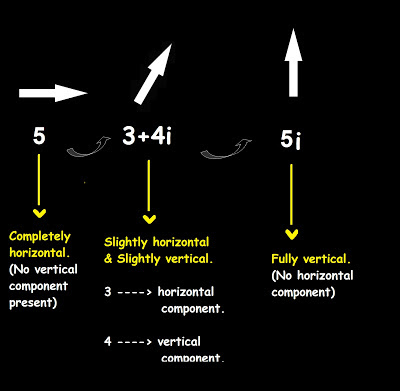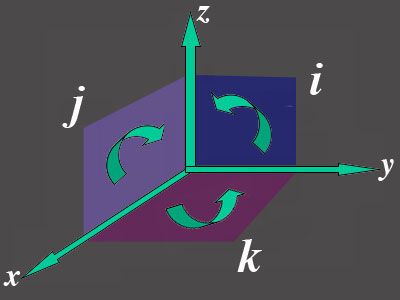Saturday, 6 August 2016

COMPLEX NUMBERS EXPLAINED INTUITIVELY AND SIMPLY

|||| The traditional number line is capable of denoting or representing only forward and backward motion. The numbers that lie on the number line such are called as REAL NUMBERS.

|||| But what if an object is moving in an inclined plane like a sphere. Now there is an added dimension to it,...right. The number line by itself cannot fully represent it. It needs help.That is where the complex plane comes into picture.

|||| The REAL PART + COMPLEX PART now  together, describe the complete motion of the object.

( )  The real component is the component which is usually the one parallel to the x-axis.
( )  The imaginary component is the one which is usually the one perpendicular to the x-axis (and parallel to the y-axis).

||||  However, even when a number like 5 gets split up into 3 + 4i when being represented on the complex plane,....the magnitude remains the same as sqrt ( 3^2 + 4^2) = 5.

||||  The numbers that can be handled by the number-line are called as REAL-NUMBERS.

||||  The numbers that cannot be handled by the number-line are called as COMPLEX-NUMBERS.

THE FAMILY OF REAL NUMBERS
||||  The family of REAL-NUMBERS include all the numbers we learn about in high school like the
Rational Numbers :- Those numbers not having a square root with them.
Integers :- Positive or Negative but those numbers not having a decimal point or any fraction involved with themselves.eg -2, -7, 8 , 5 , 2
Fractions :- You know what they are.
Irrational Numbers :- Those numbers which have a square-root attached to them. So why call them Irrational number:- I pondered about it and jokingly I came up with a reason. Maybe it would be irrational on our part to say that the car is moving at sqrt(3600) km/hr....or that my marks are sqrt ( 1600 ).

FAMILY OF COMPLEX NUMBERS.

||||  Ok, I dont know specifically of any types but i do know that i , j and k maybe used to denote the three different types in which inclination can happen.

So +i and -i will denote anticlockwise and clockwise motion repectively.
And +j and -j will denote rotations in a horizontal plane.
And +k and -k will denote rotations in a vertical plane but this time back to front or front to back.

NOW GENERALLY SPEAKING ABOUT COMPLEX NUMBERS...
|||| Complex numbers are used to denote inclination.
|||| Yeah....thats it.

|||| ‘ i ‘ as if denotes inclination. If there is no i, that means the object is completely MOVING JUST FORWARD OR BACKWARD....along a thread or a rope or a flat surface but in a line.

|||| So if the complex part is completely zero,only the real part remains....which means that there is no inclination of any kind. The object now is moving in a straight line (maybe even parallel to the x-axis many of the times).||||  Now, one could also say that Complex numbers are used to denote rotation. But then inclination and rotation are both the same.
||||  You cannot incline something without rotating it and you cannot rotate something without inclining it.

WHAT DOES 3+4i MEAN?|||||| i denotes that the object is placed at an inclination.
|||| 3 is the ‘horizontal influence’ of that object. And 4 is the ‘vertical influence’ of that object.

|||| So, what happens is, when an object gets inclined, it’s influence gets divided into two parts (components).
1) A horizontal component
2) A vertical component

|||| The vertical component is sin and the horizontal component is cos.

WHERE ALL DO COMPLEX NUMBERS COME IN REAL DAILY LIFE ? AND IN OUR EQUATIONS?

|||| Complex numbers come into our lives when one force gets divided into two branches of forces.

|||| Inclining the object is just one example where the force will get divided into two.

EXAMPLE
|||| Imagine a stick.

CASE 1: THE STICK IS COMPLETELY HORIZONTALCOMPLEX NUMBERS SOMEWHAT AN OK VIDEO||||||||||||||||||||||||||||||||||||||||||||||
|||||||||||||||||||||||||||||||||||||||||||||||||||

THE ABOVE IS A EXCERPT FROM A BOOK AVAILABLE FOR PURCHASE (5\$) AT THE FOLLOWING LINK
AVAILABLE FOR PURCHASE FOR 5\$ (INR 300) HERE (PDF)

||||||||||||||||||||||||||||||||||||||||||||||

DONATION SECTION

DONATIONS SECTION HERE

||||||||||||||||||||||||||||||||||||||||||||||||||